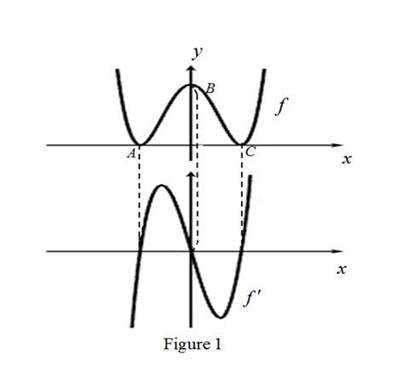# The graph of derivative of f below the graph of f.### Single Variable Calculus: Concepts...

4th Edition
James Stewart
Publisher: Cengage Learning
ISBN: 9781337687805### Single Variable Calculus: Concepts...

4th Edition
James Stewart
Publisher: Cengage Learning
ISBN: 9781337687805

#### Solutions

Chapter 2.7, Problem 4E
To determine

## To sketch: The graph of derivative of f below the graph of f.

Expert Solution

### Explanation of Solution

From the given graph, it is observed that the graph of f contains the horizontal tangents at the three points. Let the three points be A, B and C.

Note that, the value of the derivative will be zero at the point where the function has the horizontal tangent.

Thus, the graph of f will be zero at the points A, B and C.

From the point A to left, the slope of the graph f is strictly negative which implies that the derivative graph f must have a negative functional value.

From the points between A and B, the slope of the graph f is strictly positive which implies that the derivative graph f must have a positive functional value.

And, the slope of the graph f between the points B and C is strictly negative, which implies that the derivative graph f must have a negative functional value.

From point C to right, the slope of the graph f is strictly positive, which implies that the derivative graph must have a positive functional value.

Use the above information and obtain the graph of f(x) as shown below in Figure 1.From Figure1, it is observed that the function f(x) passes through the point (0,0).

### Have a homework question?

Subscribe to bartleby learn! Ask subject matter experts 30 homework questions each month. Plus, you’ll have access to millions of step-by-step textbook answers!